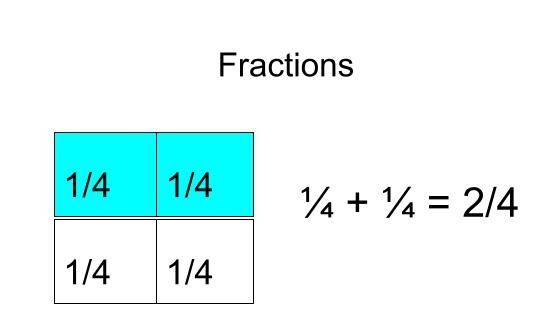Author:
Debra Berkley
Subject:
Elementary Mathematics
Material Type:
Lesson
Level:
Lower Elementary
Tags:
Lesson Plan
Creative Commons Attribution Non-Commercial Share Alike
Language:
English
Media Formats:
Text/HTML

# Fractions## Overview

Fractions - Identify, comparing, adding and subtracting fractions with common denominators.

# SUMMARY

Face to Face lesson

In this lesson students will learn what a fraction is, how to identify a fraction, and the parts of a fraction; The numerator and denominator.  Students will be able to compare fractions with <, >, =.  Students will then learn how to add and subtract fractions with common denominators.

# BACKGROUND FOR TEACHERS - CURRICULUM TIE- STANDARDS

(Strand: NUMBER AND OPERATIONS - FRACTIONS (3.NF)

Develop understanding of fractions as numbers. Denominators are limited to 2, 3, 4, 6, and 8 in third grade.

Standard 3.NF.1

Understand that a unit fraction has a numerator of one and a non-zero denominator.

1. Understand a fraction 1/b as the quantity formed by one part when a whole is partitioned into b equal parts.

2. Understand a fraction a/b as the quantity formed by a parts of size 1/b. For example: 1/4 + 1/4 + 1/4 = 3/4.

Standard 3.NF.2

Understand a fraction as a number on the number line; represent fractions on a number line diagram.

1. Represent a fraction 1/b on a number line diagram by defining the interval from 0 to 1 as the whole and partitioning it into b equal parts. Recognize that each part has size 1/b and that the endpoint of the part based at 0 locates the number 1/b on the number line.

2. Represent a fraction a/b on a number line diagram by marking off a lengths 1/b from 0. Recognize that the resulting interval has size a/b and that its endpoint locates the number a/b on the number line.

Standard 3.NF.3

Explain equivalence of fractions in special cases, and compare fractions by reasoning about their size.

1. Understand two fractions as equivalent if they are the same size, or the same point on a number line.

2. Recognize and generate simple equivalent fractions, such as 1/2 = 2/4, 4/6 = 2/3. Explain why the fractions are equivalent by using a visual fraction model, for example.

3. Express whole numbers as fractions, and recognize fractions that are equivalent to whole numbers. For example, express 3 in the form 3 = 3/1; recognize that 6/1 = 6; locate 4/4 and 1 at the same point of a number line diagram.

Compare two fractions with the same numerator or the same denominator by reasoning about their size. Recognize that comparisons are valid only when the two fractions refer to the same whole. Record the results of comparisons with the symbols >, =, or <, and justify the conclusions, for example, by using a visual fraction model.)

Cititation: UEN Utah Education Network https://www.uen.org/core/core.do?courseNum=5130

# INTENDED LEARNING OUTCOMES

1. Identify a fraction

2. Identify parts of a fraction ie. numerator and denominator

3. Compare fractions and determine which set of fractions are <, >,  or =

4. Add and subtract fractions with common denominators

TIME FRAME

60 minute lesson

# MATERIALS NEEDED

1. Chrome books for each student

2. Internet access make sure it working and students can access Nearpod and Youtube video

3. Worksheets assessments - adding and subtracting fractions

4.  Comparing fractions - fast finisher

5. How to add fractions with Common denominators

Citations: https://www.math-salamanders.com/math-fractions-worksheets.html Comparing Fractions and Adding and Subtracting Fractions - fractions free math sheets

# STEP 3 - INSTRUCTION

Into into fraction Youtube.com video Intro into Fractions

Walk through some examples of fractions with students on the whiteboard.  Example: If you had a pizza and you had 3 friends come over how many sections should your divide the pizza into? What fraction of the pizza does each person get?

Have each student play Nearpod video Fractions on their own device.

Fractions

Walk around and check for understanding and questions.

Have each student play NearPod video Comparing Fractions lesson on their own device.

Comparing Fractions lesson

Walk around and check for understanding and question

Have each student play NearPod video Comparing Fractions lesson on their own device.

Walk around and check for understanding and questions.

• Fast Finishers - Comparing fractions

• Peer buddies for struggling students

• Pull back into small groups for students that need extra help

Cititations:

Lochoff, Alex - Intro to Fractions Visually (Unit-Fractions) - https://www.youtube.com/watch?v=7lz9qfUPtPY

Kupisch, Andrew - Find the fraction of each shaded figure - https://www.youtube.com/watch?v=5KGX5Vk___I. -- Video used in Nearpod lesson that I created.

Math Antics - Comparing Fractions NearPod Lesson & Adding and Subtracting Fractions- Can be shared with Teachers - Math Antics is a series of basic math videos created by Rob and Jeremy of Math Plus Motion, LLC. https://nearpod.com/s/mathantics-A7630769

# STEP 4 - ASSESSMENT

End of lesson assessment to gage student understanding.  This is a formative assessment to check and see if students are able to add and subract fractions.  Students will need to be able to identify the numerator and denomator of a fraction.

Print needed copies of the pdf of adding and subtracting fractions

# Taking it one Step Further -Google Skills Digital Library - Probability Lesson

time: 45-90 minutes

Ask the students if they had a spinner that was split into 5 sections and if it was 2/5 likely to land on Blue, What is the probality of the spinner landing on Red?

Have the student draw the spinner.

Now how them draw a spinner where it is more likely to land on Blue.

Do the lesson with students on Probability from Google Digital Skills Library